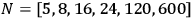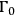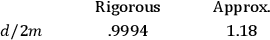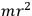# 11 The Dynamics of a Lattice Universe

R. W. Lindquist

#### DOI

10.34663/9783945561294-17

#### Citation

Lindquist, R. W. (2011). The Dynamics of a Lattice Universe. In: The Role of Gravitation in Physics: Report from the 1957 Chapel Hill Conference. Berlin: Max-Planck-Gesellschaft zur Förderung der Wissenschaften.

One considers a cosmological universe with the cosmological constant set equal to zero and asks whether it is possible to construct a closed universe which contains a finite number of mass singularities. In dealing with this problem, one works with what is essentially the Wigner-Seitz approximation carried over bodily from solid state physics. One begins by taking a space-like hypersurface or zone of influence surrounding a particle. These zones are assumed to be spheres and for the aggregate of particles each sphere is tangent to all of its nearest neighbors. Next one makes the somewhat more drastic assumption that inside each zone of influence one has a pure Schwarzschild solution. These singularities can then be fitted onto the surface of a hypersphere as shown in the figure to the left. The requirement that each zone touches its nearest neighbor at only one point requires, in view of the very high symmetry of the problem, that one takes the number of particles in the problem to be equal to the number of vertices in a regular hyperpolyhedron, i.e.,. One must now set up the tangency condition, and require that the Schwarzschild funnels shall be tangent to the hypersphere.

Fig. 11.1

This can be done in an invariant manner. One must also find how to define the hypersurfaces to begin with. This can also be done. This, with the tangency condition, gives the motion of the hypersphere. One gets from this model an expanding and contracting universe, with a cycloidal behavior.

BONDI commented that this is interesting from the point of view of the Eddington universe, admitting that this is the case with the cosmological constant not equal to zero. One can ask, if one allows for the granular structure of mass, how many granules can one allow? Of course it is your number 600.

LINDQUIST agreed and continued: In order to get an idea of how good an approximation the Schwarzschild singularities are, one can compute the maximum radius of the tangent hypersphere, for the various number of particles.

Fig. 11.2

This is shown in the above graph, in whichis the maximum radius of expansion. We have also computed the invariant distance between two Schwarzschild singularities (at the radius of maximum expansion). This has been done both rigorously - as an initial value problem - and with the above approximation. One finds the values given below:The session was concluded by WHEELER, who summarized the results presented by the various speakers.

First, from what PIRANI has said, we have gained some insight into how we may define what the measurability properties are locally of the gravitational field. The tensors and invariants he has described are at the heart of the matter. Second, as concerns the radiation problem, we would like to know what is the highest degree of symmetry one can have in a problem, and still have interesting radiation. This leads one to the question of whether, even in the cases where there is no symmetry, one has reason to expect radiation. On this score, it would be well to recall an important physical fact: that the gravitational field of a point charge has close analogies to the electric field. One knows that there is a certain linear approximation to the field equations similar in nature to the electromagnetic equations, so that if a mass is accelerating, one finds it produces radiation similar to the electromagnetic radiation of an accelerating charge. On this account, one expects gravitational radiation. Using this analogy, Einstein was able to calculate the rate of radiation from a double star. The issue which SCHILD brought up is one quite different from the double star radiation, in that the system is time-nonsymmetric. He invites us to consider the radiation produced in a gravitational scattering process. The acceleration of the particle as it zooms by the stationary one will give radiation at large distances, but the recoil acceleration of the particle which was initially at rest will produce a wave of nearly the opposite phase of the first, so that one expects the rate of radiation to be proportional to something analogous to the moment of inertia of the double star system. This means that the damping of this system depends on some complicated geometric concept like. Therefore, if one hopes to get a proper answer to the question of how to describe the “frictional force” between the two masses, one must find some quite general way of describing invariantly that region of space in which the particle undergoes its acceleration.